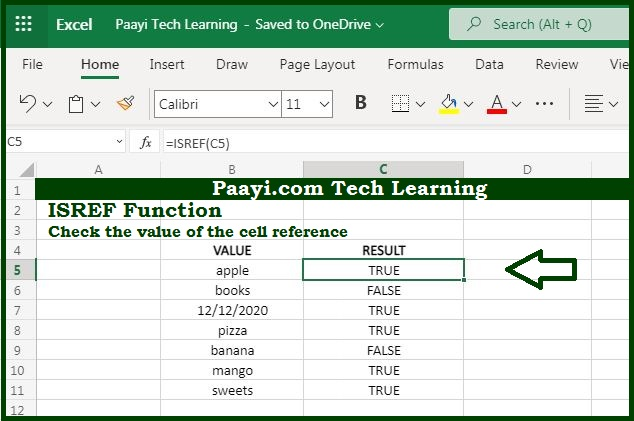# Learn How to Use Microsoft Excel ISREF Function

Written by | 0 Comments | 688 Views

In this article, you will learn how to use the Microsoft Excel ISREF function and its prime function in Microsoft Excel. You will also get to know the Microsoft Excel ISREF function return value and syntax with the help of some examples.

Microsoft Excel ISREF Function

The main function of the Microsoft Excel ISREF function is to test for a reference. That means with the help of the ISREF function you can able to get the return value as the TRUE if the cell is having reference, and FALSE if it is not. You can also use the ISREF function to check whether the cell contains valid reference or not.

Return Value of ISREF Function

The return value will be logical TRUE or FALSE.

Syntax of ISREF Function

=ISREF(value)

Where the arguments:

• value: It is the value to check.

## How to Use Microsoft Excel ISREF Function?The main function of the Microsoft Excel ISREF function is to test a reference. That means with the help of the ISREF function you can able to get the return value as the TRUE if the cell contains the reference, and FALSE if it does not

It should be noted that:

• The ISREF function is the part of the "IS" function that returns the logical value TRUE or FALSE.